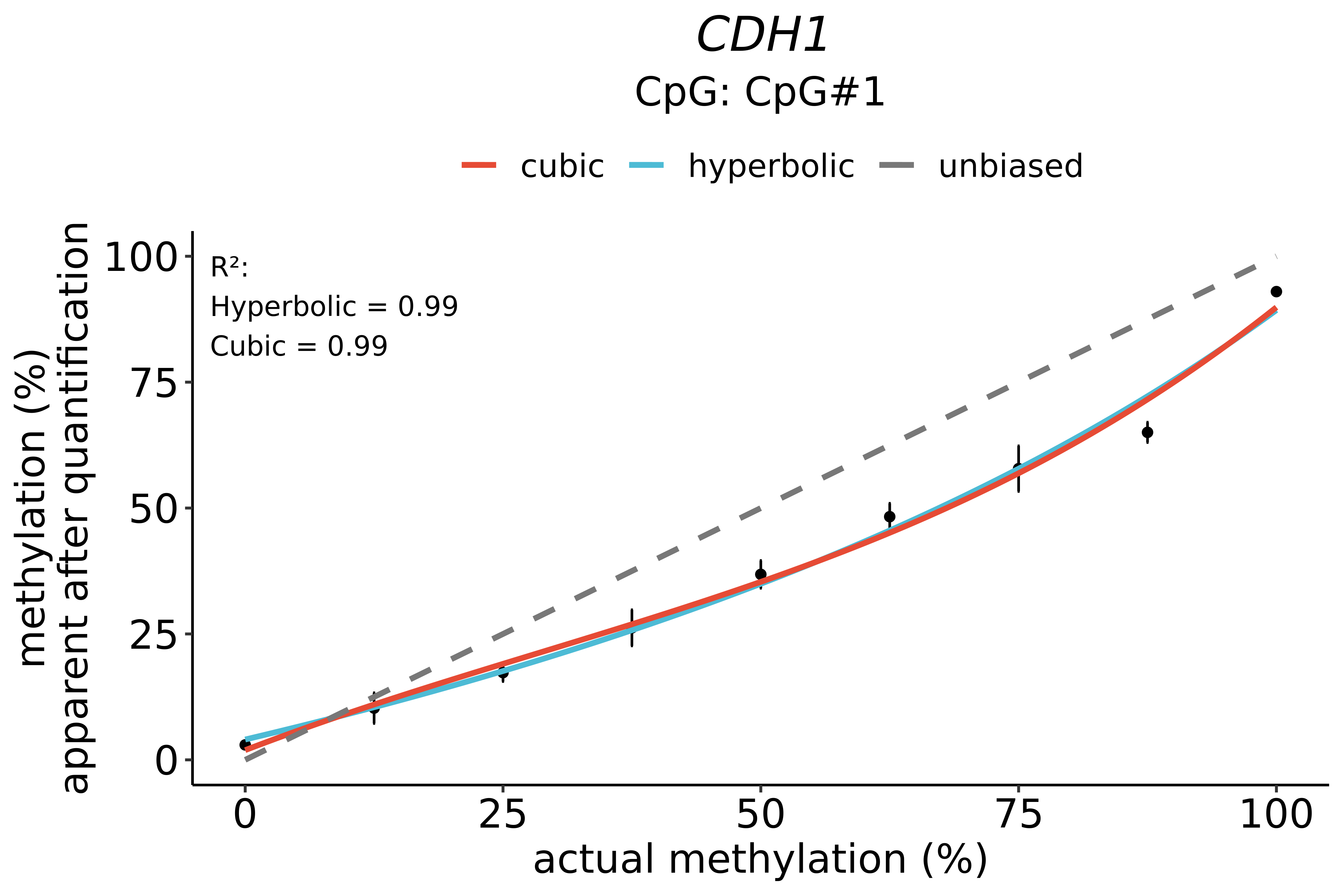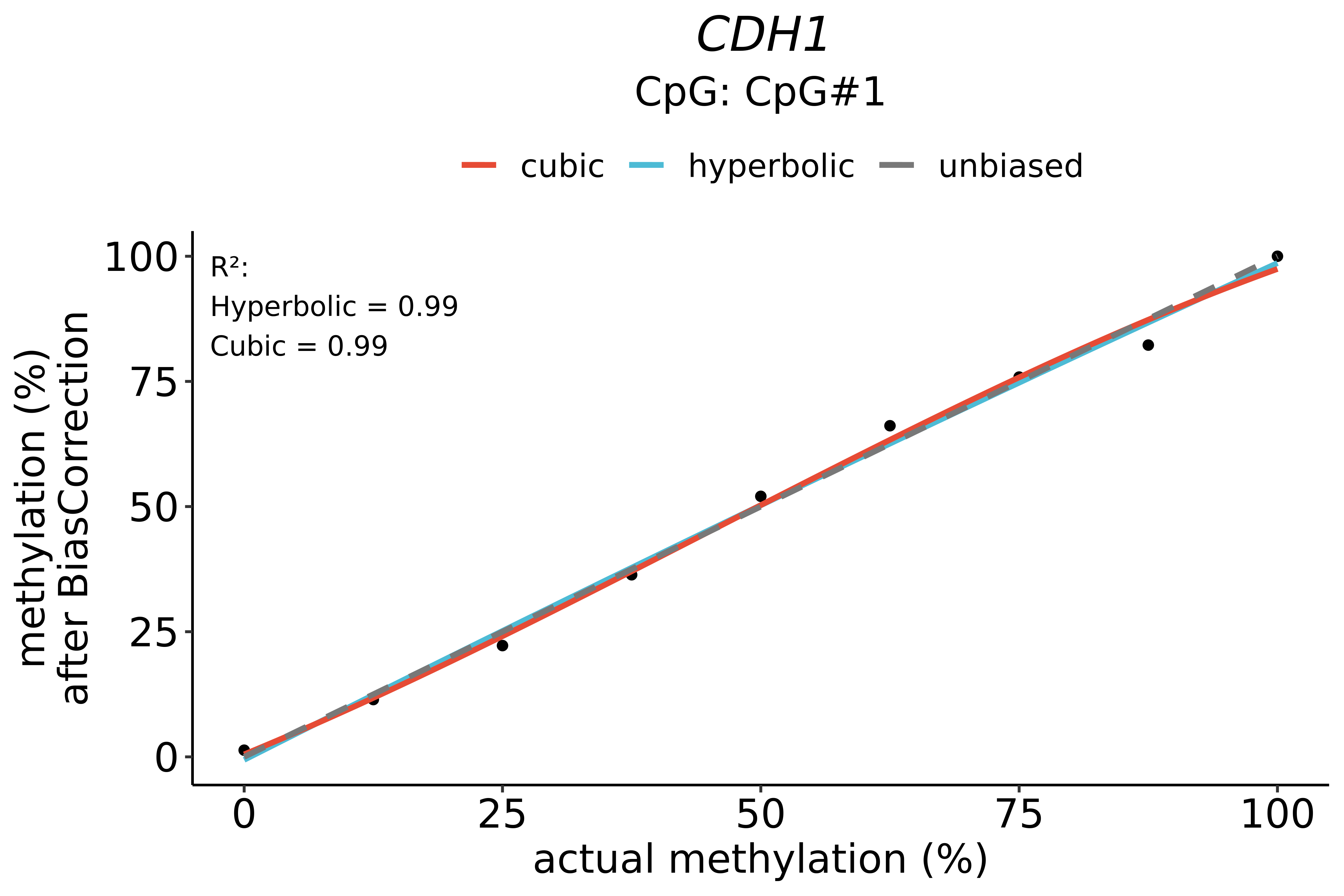# rBiasCorrection

#### 2020-12-17

library(rBiasCorrection)

# Introduction

rBiasCorrection is the R implementation of the algorithms described by Moskalev et. al in the research article ‘Correction of PCR-bias in quantitative DNA methylation studies by means of cubic polynomial regression’, published 2011 in Nucleic acids research, Oxford University Press (DOI: https://doi.org/10.1093/nar/gkr213).

# Setup the prerequisites

First of all, some variables need to be defined. These include:

• the path to the experimental file, including its filename
• the path to the calibration file, including its filename
• samplelocusname: the name of the sample or locus under investigation
• the seed argument should be set for reproducibility
• plotdir: a folder, where the resulting plots should be stored
• csvdir: a folder, where the resulting tables should be stored
plotdir <- paste0(tempdir(), "/png/")
csvdir <- paste0(tempdir(), "/csv/")
dir.create(plotdir)
#> Warning in dir.create(plotdir): '/tmp/RtmpvUIchZ/png' already exists
dir.create(csvdir)
#> Warning in dir.create(csvdir): '/tmp/RtmpvUIchZ/csv' already exists

samplelocusname <- "CDH1"
seed <- 1234

For demonstration purposes, we will here correct experimental biases in only one CpG site. The example data is included in this R package.

experimental <- paste0(tempdir(), "/experimental_data.csv")
calibration <- paste0(tempdir(), "/calibration_data.csv")

# Conduct the correction of experimental biases

The aforementioned variables can now be passed to the function rBiasCorrection::biascorrection in order to calculate the bias-corrected values of the experimental data.

rBiasCorrection::biascorrection(
experimental = experimental,
calibration = calibration,
samplelocusname = samplelocusname,
plotdir = plotdir,
csvdir = csvdir,
seed = seed,
parallel = FALSE
)

# Background information

First of all, a preprocessing step is performed. During this step, all requirements of the input files are checked (please find further information of the specific file requirements in the FAQ). Furthermore, the mean methylation percentages of all CpG sites are calculated for every provided file and stored in a new column rowmeans.

Biases are calculated using two regression algorithms: hyperbolic and cubic polynomial regression. With the default settings, the general forms of hyperbolic and cubic polynomial equations are used. However, an experimental feature exists, which can be accessed by using the argument minmax = TRUE. These special regression equations are data-dependent, assuming, that the minima and maxima of the provided calibration data are not biased at all (e.g. 100% actual methylation corresponds to 100% observed methylation).

# General regression equations

Hyperbolic equation:

$\begin{equation} y = \frac{(a * x) + b}{x + d} \end{equation}$

Cubic polynomial equation:

$\begin{equation} y = a * x^3 + b * x^2 + c * x + d \end{equation}$

# Data dependent regression equations (experimental feature)

• m0: the actual minimum of the calibration data
• m1: the actual maximum of the calibration data
• y0: the observed minimum of the calibration data (after quantification)
• y1: the observed maximum of the calibration data (after quantification)

Hyperbolic equation:

$\begin{equation} y = \frac{((b * y1) - y0) * (x - m0) + (m1 - m0) * y0}{(b - 1) * (x - m0) + (m1 - m0)} \end{equation}$

Cubic polynomial equation:

$\begin{equation} y = a * (x - m0)^3 + b * (x - m0)^2 + [\frac{y1 -y0}{m1 - m0} - a * (m1 - m0)^2 - b * (m1 - m0)] * (x - m0) + y0 \end{equation}$

# Selection of the correction algorithm

The correction algorithm to correct the biases can be chosen by setting the argument correct_method to either ‘hyperbolic’ or ‘cubic’. If using the default setting ‘best’, the regression method will be selected for each CpG site based on the most appropriate method, specified in the selection_method argument.

The selection_method argument can be either ‘SSE’ (the default setting) or ‘RelError’. By using ‘SSE’, the error sum of squares (SSE) is calculated for each CpG site for both regression methods. The regression method resulting in a lower (better) SSE is then subsequently used to correct the biases of the corresponding experimental data. “RelError” selects the regression method based on the theoretical relative error after correction. This metric is calculated by correcting the calibration data with both the hyperbolic regression and the cubic regression and using them again as input data to calculate the ‘goodness of fit’-metrics.

# Outputs and Results

Resulting tables and plots can now be found in the directories specified in csvdir and plotdir.

All file names are prefixed with the name, specified in samplelocusname. The tables are stored as CSV-files and include a timestamp in their file name. The plots are stored as PNG-files. Their size can be specified with the arguments plot_height, plot_width and plot_textsize, which can optionally be passed to the function rBiasCorrection::biascorrection.

The following tables are stored:

• [name]corrected_values[timestamp].csv: the bias corrected experimental data (this is the final results table of the samples under investigation)
• [name]regression_stats[timestamp].csv: the regression parameters calculated for each CpG site, including goodness-of-fit metrics
• [name]corrected_calibrations_h[timestamp].csv: the calibration data, which has been bias corrected using the hyperbolic regression parameters for all CpG sites of an interrogated locus
• [name]corrected_calibrations_c[timestamp].csv: the calibration data, which has been bias corrected using the cubic regression parameters for all CpG sites of an interrogated locus
• [name]corrected_regression_stats_h[timestamp].csv: the regression parameters calculated, using the [name]corrected_calibrations_h[timestamp].csv-file as input data
• [name]corrected_regression_stats_c[timestamp].csv: the regression parameters calculated, using the [name]corrected_calibrations_c[timestamp].csv-file as input data

Regression statistics:

The regression statistics table shows the regression parameters of the hyperbolic and the cubic polynomial regression.

• Column 1 presents the CpG site’s ID.
• Column 2 contains the mean of the relative absolute errors for every interrogated CpG site.
• Columns 3-9 comprise the sum of squared errors of the hyperbolic regression (‘SSE [h]’) and the coefficients of the hyperbolic equation that describes the hyperbolic regression curves for the respective CpG sites.
• Columns 10-15 summarize the sum of squared errors of the cubic polynomial regression (‘SSE [c]’) and the coefficients of the cubic polynomial equations.
• The rows highlighted with a green background color indicate the regression method (hyperbolic or cubic polynomial) that is suggested by BiasCorrector for correcting data. This automatic choice of the regression method relies on either minimizing the value of SSE (the default setting) or minimizing the average relative error as selected by the user in the Settings tab.
filename <- list.files(csvdir)[grepl("regression_stats_[[:digit:]]", list.files(csvdir))]
knitr::kable(reg_stats[,1:9])
Name relative_error SSE_hyperbolic R2_hyperbolic a_hyperbolic b_hyperbolic d_hyperbolic b1_hyperbolic s_hyperbolic
CpG#1 22.91426 77.3425 0.9884756 -108.568 -937.7194 -232.0571 0.5690716 4.07579
row_means 22.91426 77.3425 0.9884756 -108.568 -937.7194 -232.0571 0.5690716 4.07579
knitr::kable(reg_stats[,11:16])
SSE_cubic R2_cubic a_cubic b_cubic c_cubic d_cubic
71.00228 0.9894303 6.53e-05 -0.0055807 0.7840619 1.931827
71.00228 0.9894303 6.53e-05 -0.0055807 0.7840619 1.931827

# Regression plots

Calibration plots:

The calibration plots show two calibration curves for each CpG site: the hyperbolic and the cubic polynomial regression curve.

knitr::include_graphics(paste0(plotdir, "CDH1_CpG1.png"))Corrected calibration plots and error plots:

Furthermore, corrected calibration plots and error plots are drawn. The corrected calibration plots show the theoretical regression curve after bias correction. There is one plot for each regression method and CpG site. Additionally, error plots show the efficiency of the bias correction by presenting the relative errors before and after correction.